# Definition of Hybridization

What is Hybridization?

Hybridization happens when atomic orbitals mix to form a new atomic orbital. The new orbital can hold the same total number of electrons as the old ones. The properties and energy of the new, hybridized orbital are an 'average' of the original unhybridized orbitals.

The concept of hybridization was introduced because it was the best explanation for the fact that all the C - H bonds in molecules like methane are identical.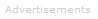Example

In their ground state, carbon atoms naturally have electron configuration 1s2 2s2 2p2.

The four outermost electrons, i.e. those in the 2s and 2p sublevels are available to form chemical bonds with other atoms.

The 2s orbital is capable of holding up to two electrons, and there are three 2p orbitals, each capable of holding up to two electrons, which means the 2p orbitals can hold up to six electrons.

Individually, these electron orbitals look something like this. (Each is centered on carbon's nucleus and the p orbitals make angles of 90° with one another.)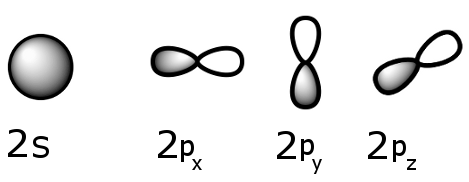The two lobes of a p orbital, one shaded, the other not, represent different phases of the wave function Ψ.

When a p orbital meets another orbital, their phases can interfere constructively or destructively. This is shown in the schematic below: an s orbital and a p orbital come together and hybridize. Like sign phases interfere constructively. There is destructive interference if the signs of the phases are different.

The end result is an sp hybrid orbital.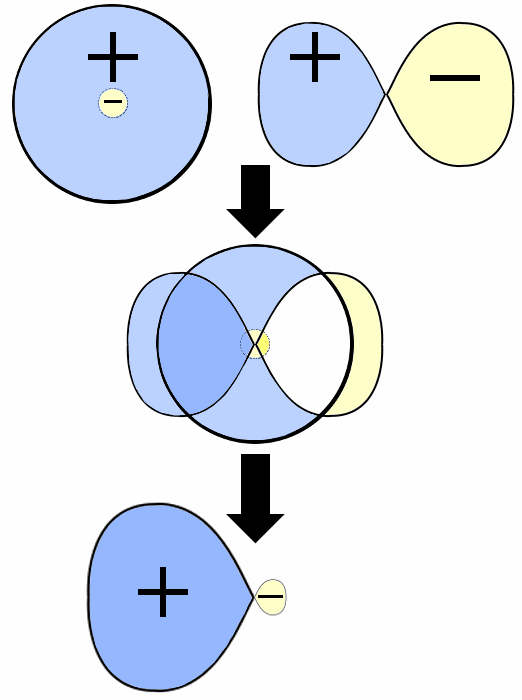Taking it further, the 2s orbital and the three 2p orbitals can hybridize to form a new hybrid orbital, called sp3, which is capable of holding up to eight electrons.

The sp3 hybrid orbital, which is the sum of the original 2s and 2p orbitals, looks something like this:sp3 hybrid orbitals have a tetrahedral shape - each orbital makes an angle of 109.5° with the others. This angle maximizes the distance between the orbital limbs, which is natural given that the electrons in each of the limbs repel one another.

The shape of molecules like methane, CH4, with bond angles of 109.5°, is consistent with sp3 hybridization of carbon atoms.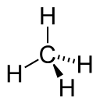Here is an energy level diagram showing how electron energies change in hybridization.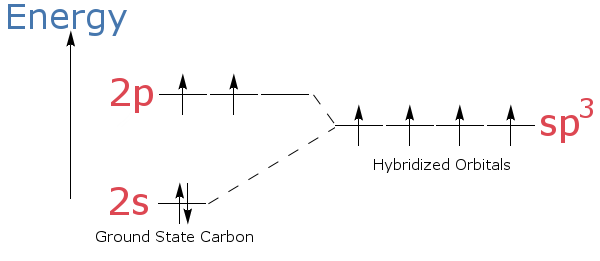Search the Dictionary for More Terms